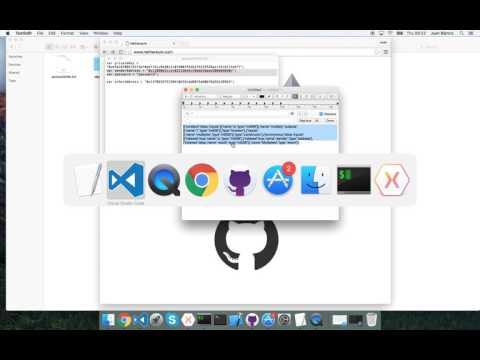# Calls, transactions, events, filters and topics

The previous guide covered how to deploy and call a contract, this guide will delve a bit deeper into calling contracts, transactions, events and topics.

## Videos

This hands-on demo covers the steps provided in this guide for calls, transactions, events, filters and topics## The test contract

The following smart contract is an updated version of the “multiply” contract from the previous guide:

contract test {

int _multiplier;
event Multiplied(int indexed a, address indexed sender, int result );

function test(int multiplier) {
_multiplier = multiplier;
}

function multiply(int a) returns (int r) {
r = a * _multiplier;
Multiplied(a, msg.sender, r);
return r;
}
}

The smart contract now includes an Event called “Multiplied”. The event will store on the log the original parameter for multiplication “a”, the address of the “sender” and the “result” of the multiplication. The parameter “a” and the “sender” address are both indexed so we can create specific filters for those two values using topics.

## Deploying the contract

As per the previous guide, we can deploy the contract as follows:

    var senderAddress = "0x12890d2cce102216644c59daE5baed380d84830c";

var multiplier = 7;

var web3 = new Web3.Web3();

Assert.True(unlockResult);

var transactionHash = await web3.Eth.DeployContract.SendRequestAsync(abi, byteCode, senderAddress, new HexBigInteger(900000), multiplier);
var receipt = await MineAndGetReceiptAsync(web3, transactionHash);


## The multiply transaction

When performing a call, we are either retrieving data which is stored in the smart contract state or we are performing an action (i.e multiplication), calls are not transactions which are verified through the blockchain consensus.

Submitting a transaction to perform a function operation in a smart contract does not return the result of the operation, events can be used to retrieve information or we can inspect the state of the smart contract by using function calls.

    var contractAddress = receipt.ContractAddress;

var multiplyFunction = contract.GetFunction("multiply");

receipt = await MineAndGetReceiptAsync(web3, transactionHash);


Using the contract address from deploying the transaction we can create an instance of a contract object and the function “multiply”.

The function object simplifies submitting transactions in the same way as calls. As per the example above we just need to include the “senderAddress” which will be charged the gas associated with the operation together with the parameters for the function operation.

There is also the option to specify the gas or include an Ether value as part of the transaction.

On the example, we have submitted 2 transactions to perform a multiplication for 7 and 8 respectively, and are waiting for the transaction to be mined on our private test chain.

## Events, filters and topics

### Creating events and filters

Events are defined as part of the abi, and similarly to the functions we can get events using our contract instance.

 var multiplyEvent = contract.GetEvent("Multiplied");


The event object allows to create filters in order to retrieve the information stored on the log.

We can create filters that retrieve all the event logs

var filterAll = await multiplyEvent.CreateFilterAsync();


Or for an specific topic

var filter7 = await multiplyEvent.CreateFilterAsync(7);


In the example above we are retrieving the logs in which the multiply parameter is 7, because the input parameter for the multiplication is marked as indexed, we can filter for that topic.

In a similar way, we can filter the sender address as it is also marked as indexed, but if we wanted to filter for that specific topic we will use the second parameter when creating the filter.

var filterSender = await multiplyEvent.CreateFilterAsync(null, senderAddress);


### Event DTO

Event data transfer objects allows to simply decode all the event parameters into a transfer object, in a similar way as we will deserialise a Json object.

 public class MultipliedEvent
{
[Parameter("int", "a", 1, true)]
public int MultiplicationInput {get; set;}

public string Sender {get; set;}

[Parameter("int", "result", 3, false)]
public int Result {get; set;}

}

In the example above, the MultipliedEvent properties have been “mapped” with custom parameter attributes to the event parameters. Each parameter specifies the original type, name, order and if is indexed or not. As we can see, types like address are decoded into strings and in our scenario we are safe to decode int256 to int32 but if not known, the final type BigInteger would have been a better option.

### Retrieving the events and logs

Using the filters we have already created, we can retrieve the logs and events.

 var log = await multiplyEvent.GetFilterChanges<MultipliedEvent>(filterAll);
var log7 = await multiplyEvent.GetFilterChanges<MultipliedEvent>(filter7);


Above we are using GetFilterChanges, which can be used to retrieve any logs that matches our criteria since the filter was created or since the last time we tried to retrieve the changes. Other option would have been to use GetAllChanges using the FilterInput.

### The final code

All the source code can be found under CallTransactionEvents in the Tutorials solution

    public async Task ShouldBeAbleCallAndReadEventLogs()
{

var multiplier = 7;

var web3 = new Web3.Web3();

Assert.True(unlockResult);

var transactionHash = await web3.Eth.DeployContract.SendRequestAsync(abi, byteCode, senderAddress, new HexBigInteger(900000), multiplier);
var receipt = await MineAndGetReceiptAsync(web3, transactionHash);

var multiplyFunction = contract.GetFunction("multiply");

var multiplyEvent = contract.GetEvent("Multiplied");

var filterAll = await multiplyEvent.CreateFilterAsync();
var filter7 = await multiplyEvent.CreateFilterAsync(7);

receipt = await MineAndGetReceiptAsync(web3, transactionHash);

var log = await multiplyEvent.GetFilterChanges<MultipliedEvent>(filterAll);
var log7 = await multiplyEvent.GetFilterChanges<MultipliedEvent>(filter7);

Assert.Equal(2, log.Count);
Assert.Equal(1, log7.Count);
Assert.Equal(7, log7.Event.MultiplicationInput);
Assert.Equal(49, log7.Event.Result);
}

//event Multiplied(int indexed a, address indexed sender, int result );

public class MultipliedEvent
{
[Parameter("int", "a", 1, true)]
public int MultiplicationInput {get; set;}

public string Sender {get; set;}

[Parameter("int", "result", 3, false)]
public int Result {get; set;}

}

public async Task<TransactionReceipt> MineAndGetReceiptAsync(Web3.Web3 web3, string transactionHash){

var miningResult = await web3.Miner.Start.SendRequestAsync(6);
Assert.True(miningResult);

var receipt = await web3.Eth.Transactions.GetTransactionReceipt.SendRequestAsync(transactionHash);

while(receipt == null){